Ex.3.7 (Optional)

Chapter 3 Class 10 Pair of Linear Equations in Two Variables
Serial order wise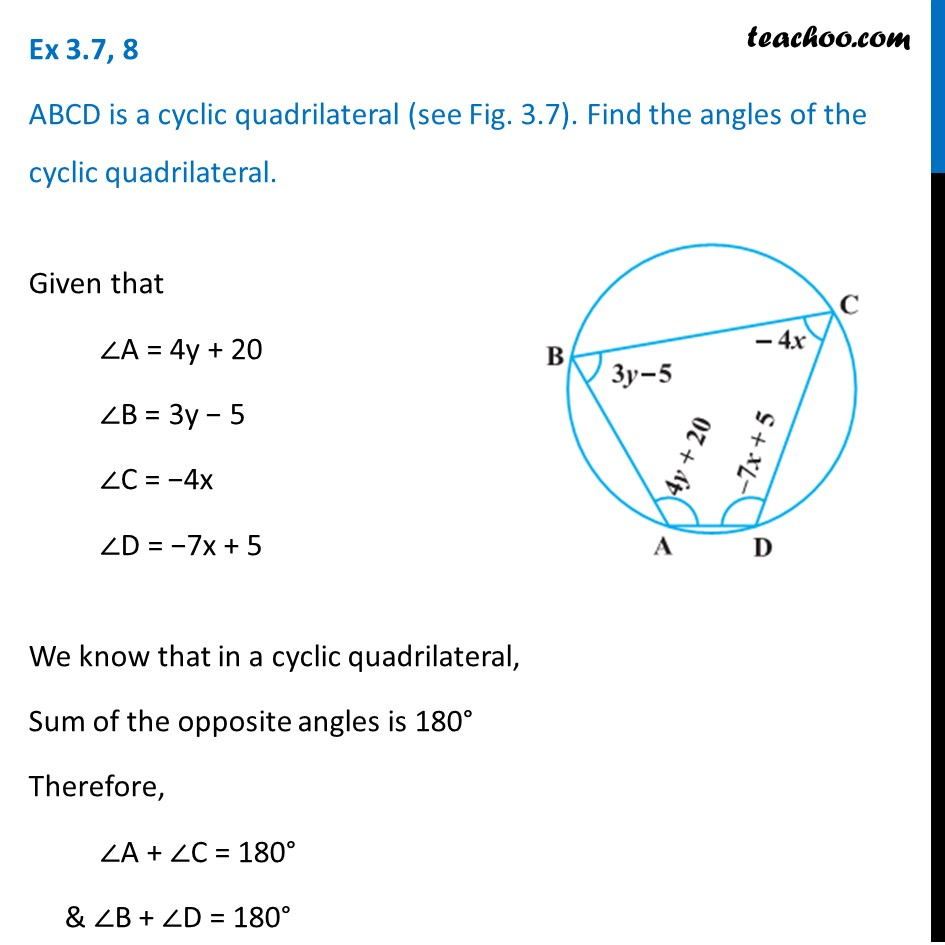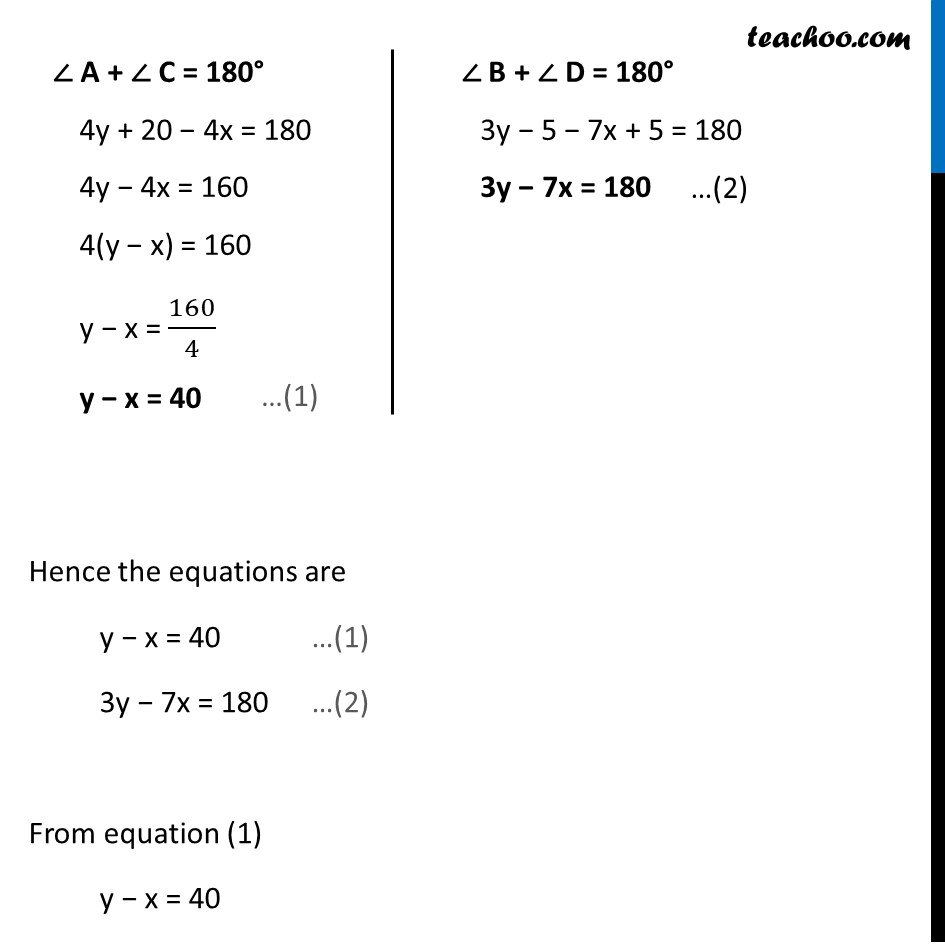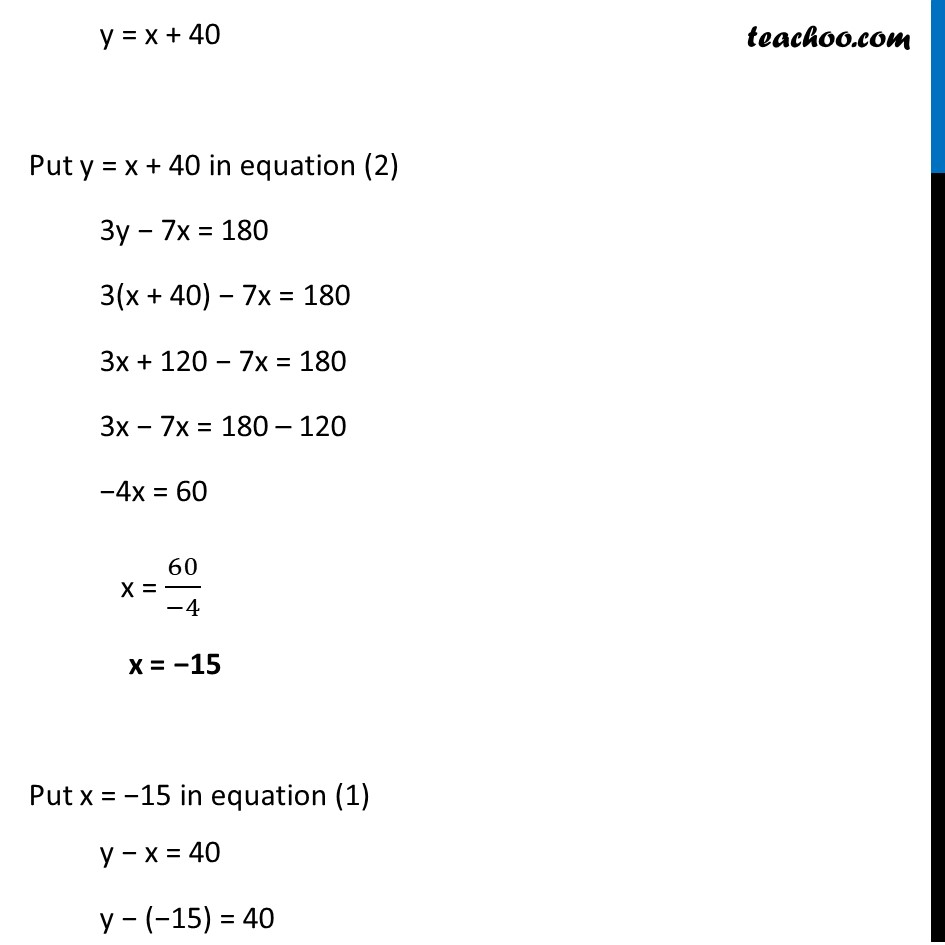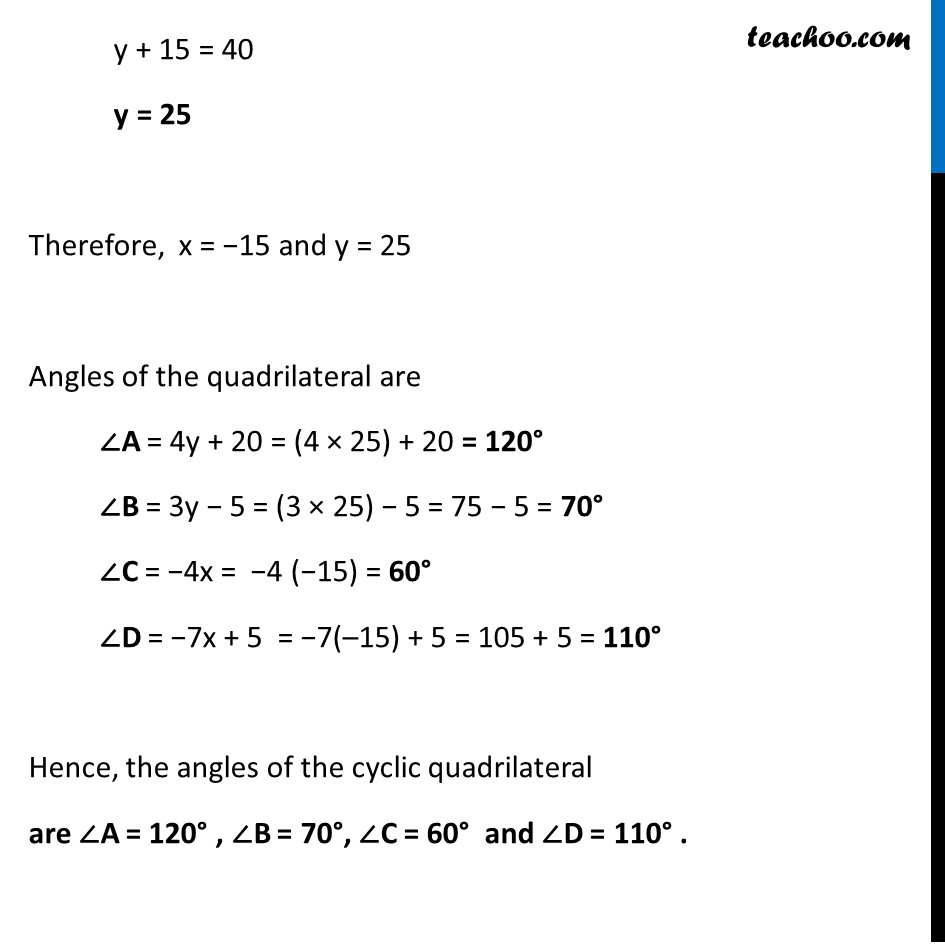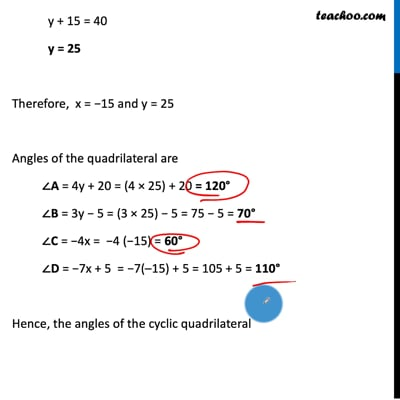This video is only available for Teachoo black users

Introducing your new favourite teacher - Teachoo Black, at only ₹83 per month

### Transcript

Ex 3.7, 8 ABCD is a cyclic quadrilateral (see Fig. 3.7). Find the angles of the cyclic quadrilateral. Given that ∠A = 4y + 20 ∠B = 3y − 5 ∠C = −4x ∠D = −7x + 5 We know that in a cyclic quadrilateral, Sum of the opposite angles is 180° Therefore, ∠A + ∠C = 180° & ∠B + ∠D = 180° ∠ A + ∠ C = 180° 4y + 20 − 4x = 180 4y − 4x = 160 4(y − x) = 160 y − x = 160/4 y − x = 40 ∠ B + ∠ D = 180° 3y − 5 − 7x + 5 = 180 3y − 7x = 180 Hence the equations are y − x = 40 …(1) 3y − 7x = 180 …(2) From equation (1) y − x = 40 y = x + 40 Put y = x + 40 in equation (2) 3y − 7x = 180 3(x + 40) − 7x = 180 3x + 120 − 7x = 180 3x − 7x = 180 – 120 −4x = 60 x = 60/(−4) x = −15 Put x = −15 in equation (1) y − x = 40 y − (−15) = 40 y + 15 = 40 y = 25 Therefore, x = −15 and y = 25 Angles of the quadrilateral are ∠A = 4y + 20 = (4 × 25) + 20 = 120° ∠B = 3y − 5 = (3 × 25) − 5 = 75 − 5 = 70° ∠C = −4x = −4 (−15) = 60° ∠D = −7x + 5 = −7(–15) + 5 = 105 + 5 = 110° Hence, the angles of the cyclic quadrilateral are ∠A = 120° , ∠B = 70°, ∠C = 60° and ∠D = 110° .